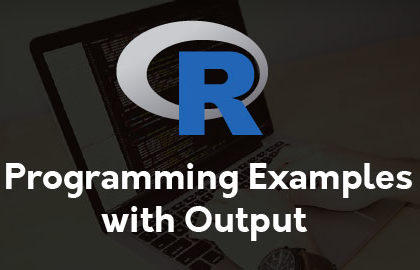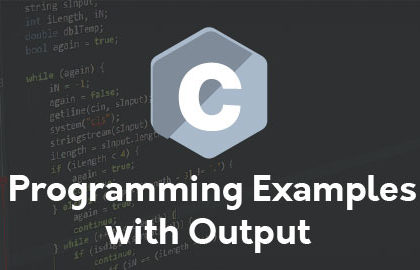# Interview Topics

#### COBOL Interview Questions and AnswersTensorFlow is an open source framework, by Google for creating deep learning models. Deep Learning is one of several categories of machine learning (ML) models that use multi-layer neural networks. TensorFlow is cross-platform. It runs on nearly everything: GPUs and CPUs—including mobile and embedded...

#### TensorFlow Interview Questions and Answers 2019

TensorFlow is an open source framework, by Google for creating deep learning models. Deep Learning is one of several categories of machine learning (ML) models that use multi-layer neural networks. TensorFlow is cross-platform. It runs on nearly everything: GPUs and CPUs—including mobile and embedded...Here,You can Learn Basic R Programming Examples with Output for Freshers R Programming: Once you have R environment setup, then it’s easy to start your R command prompt by just typing the following command at your command prompt- \$R This will launch R interpreter...

#### Basic R Programming Examples With Output

Here,You can Learn Basic R Programming Examples with Output for Freshers R Programming: Once you have R environment setup, then it’s easy to start your R command prompt by just typing the following command at your command prompt- \$R This will launch R interpreter...Here,You can learn Top Most asked C Programming Examples with Output for Freshers. C Programming Example: 1. Write a C Program to Hello World 2. Write a C program to add two integers 3. Write a C program to addition, subtraction, multiplication,     ...

#### Basic C Programming Examples With Output

Here,You can learn Top Most asked C Programming Examples with Output for Freshers. C Programming Example: 1. Write a C Program to Hello World 2. Write a C program to add two integers 3. Write a C program to addition, subtraction, multiplication,     ...

The least common multiple (L.C.M.) of two numbers is the smallest positive integer that is perfectly divisible by the two given numbers. For example, the L.C.M. of 12 and 14 is 84. Program: lcm <- function(x, y) { # choose the greater number if(x...

#### Write a R Program to Find LCM

The least common multiple (L.C.M.) of two numbers is the smallest positive integer that is perfectly divisible by the two given numbers. For example, the L.C.M. of 12 and 14 is 84. Program: lcm <- function(x, y) { # choose the greater number if(x...

The highest common factor (H.C.F) or greatest common divisor (G.C.D) of two numbers is the largest positive integer that perfectly divides the two given numbers. For example, the H.C.F of 12 and 14 is 2. Program: hcf <- function(x, y) { # choose the...

#### Write a R Program to Find GCD

The highest common factor (H.C.F) or greatest common divisor (G.C.D) of two numbers is the largest positive integer that perfectly divides the two given numbers. For example, the H.C.F of 12 and 14 is 2. Program: hcf <- function(x, y) { # choose the...